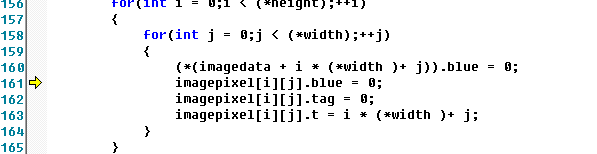C++,为一个二维数组赋值，为什么到一半就停止了？

int preprocessing(char strFile[],IMAGEDATA imagedata,PIXEL **imagepixel,long *wid,long *hei)
{
FILE *fpi;
imagedata= NULL;//动态分配存储原图片的像素信息的二维数组
long *width,*height;//新定义一个像素结构体二维数组
width=wid;height=hei;
fpi=fopen(strFile,"rb");

if(fpi != NULL){

//先读取文件类型

WORD bfType;

if(0x4d42!=bfType)

{

cout<<"the file is not a bmp file!"<<endl;

return NULL;

}

//读取bmp文件的文件头和信息头

//读取调色板

for(unsigned int nCounti=0;nCounti<strInfo.biClrUsed;nCounti++)

{

}

width = &strInfo.biWidth;

height = &strInfo.biHeight;

//图像每一行的字节数必须是4的整数倍

*width = ((*width) * sizeof(IMAGEDATA) + 3) / 4 * 4;
*wid=*width;
*hei=*height;
imagedata = (IMAGEDATA
)malloc((*width) * (*height) * sizeof(IMAGEDATA));

imagepixel=(PIXEL**)malloc(sizeof(PIXEL*)*(*height));
for(int w=0;w<(*height);w++)
{
imagepixel[w]=(PIXEL*)malloc(sizeof(PIXEL*)*(*width));
}
//初始化原始图片的像素数组以及新构造的像素数组

for(int i = 0;i < (*height);++i)

{

for(int j = 0;j < (*width);++j)

{

(*(imagedata + i * (*width )+ j)).blue = 0;
imagepixel[i][j].blue = 0;//系统提示这一句；
imagepixel[i][j].tag = 0;
imagepixel[i][j].t = i * (*width )+ j;
}

}

//fseek(fpi,54,SEEK_SET);

//读出图片的像素数据

fclose(fpi);
for(i = 0;i <(*height);++i)

{

for(int j = 0;j < (*width);++j)

{

imagepixel[i][j].blue=(
(imagedata + i * (*width) + j)).blue;
}
}

processingpixel(imagedata,imagepixel,width,height);//对像素操作

}

else

{

cout<<"file open error!"<<endl;

return NULL;

}
fclose(fpi);
afterprocessing(imagedata,width,height);
return 0;
}